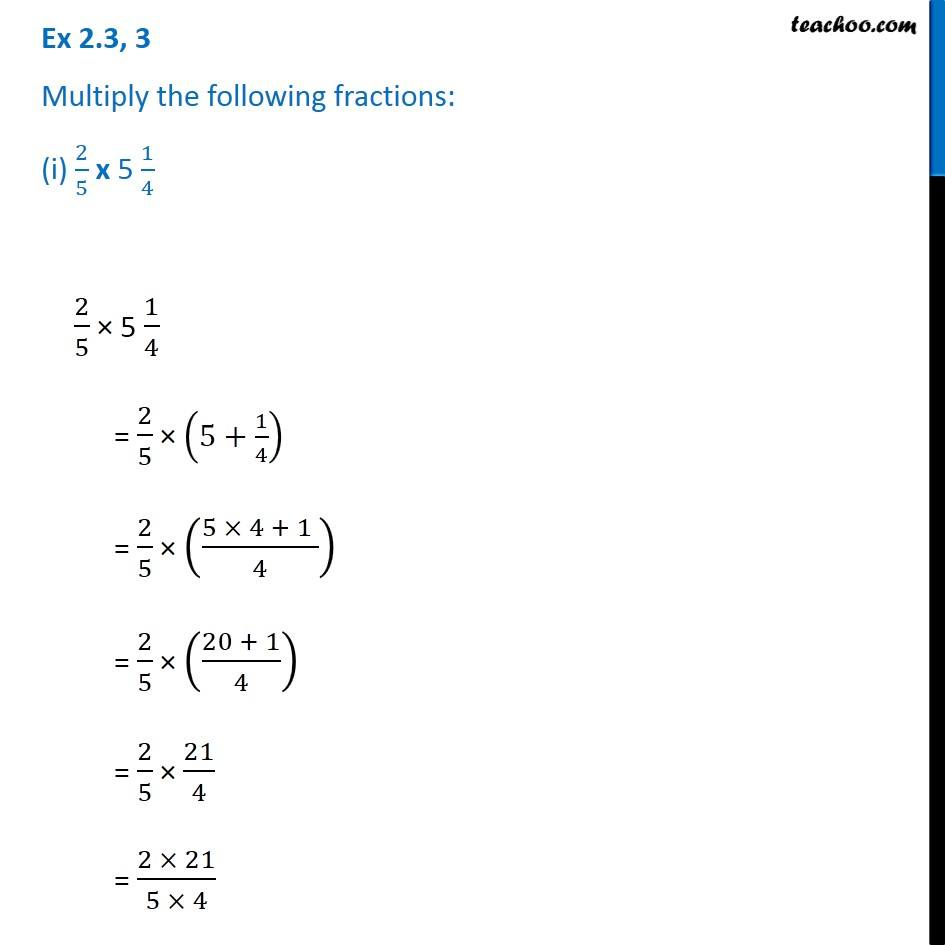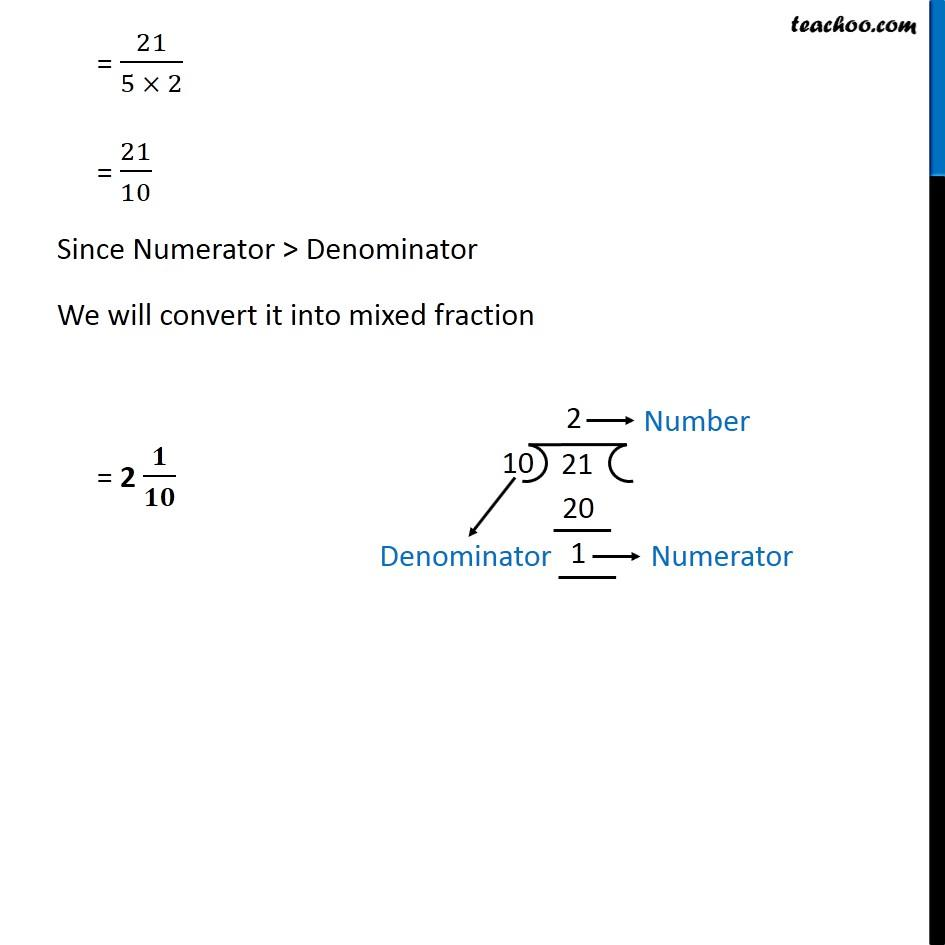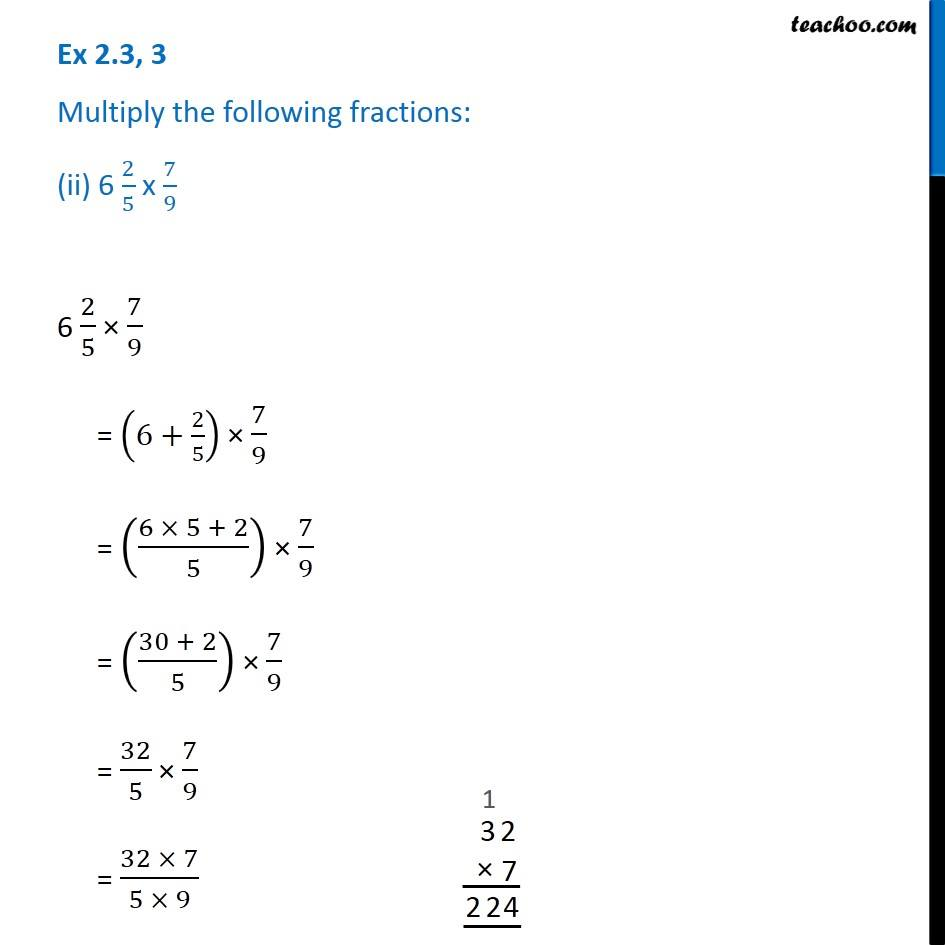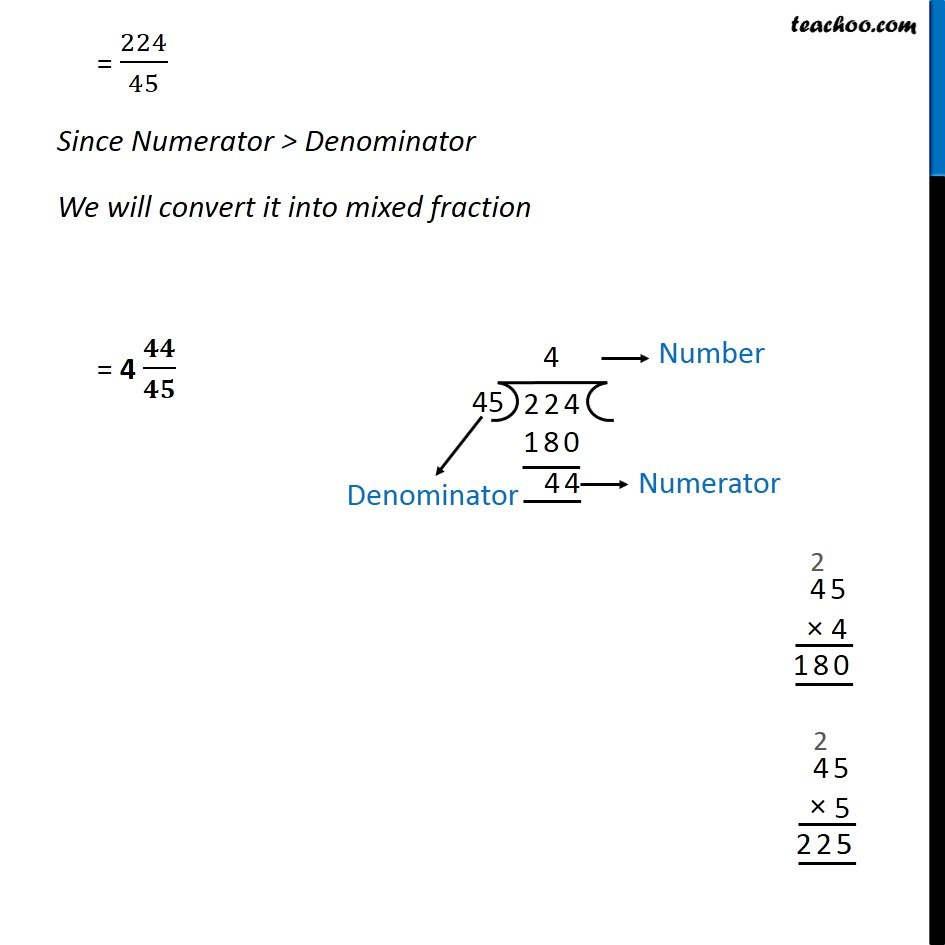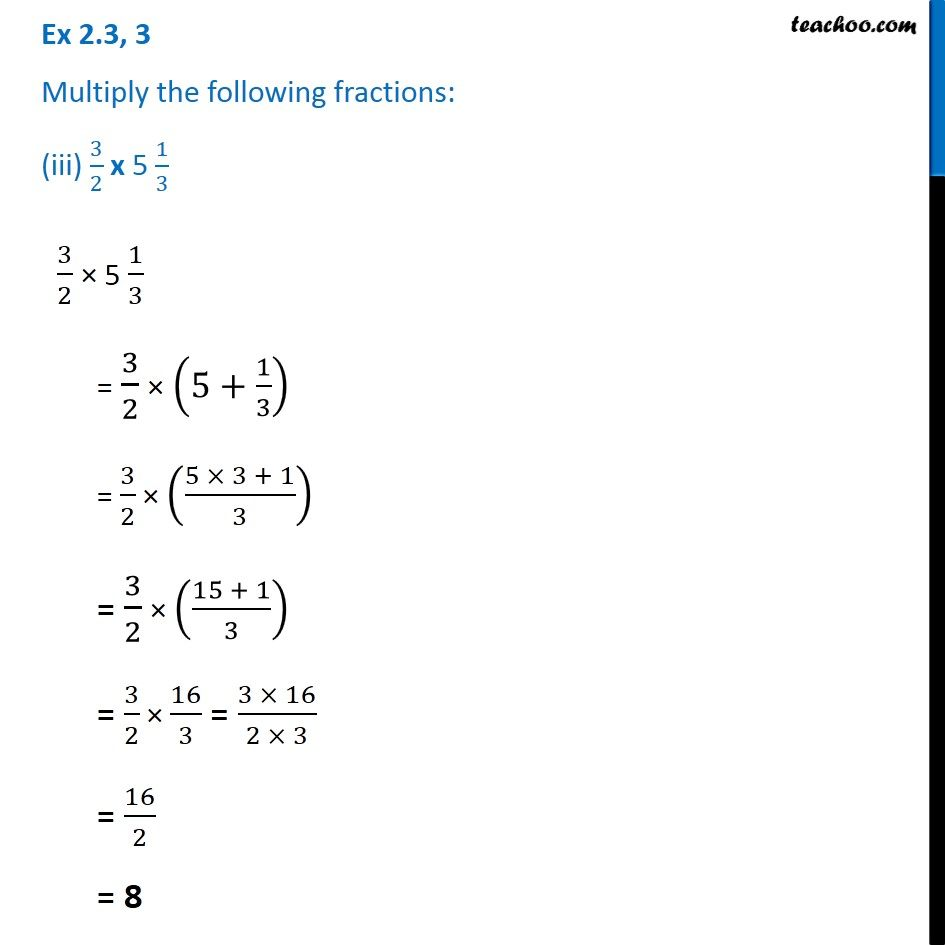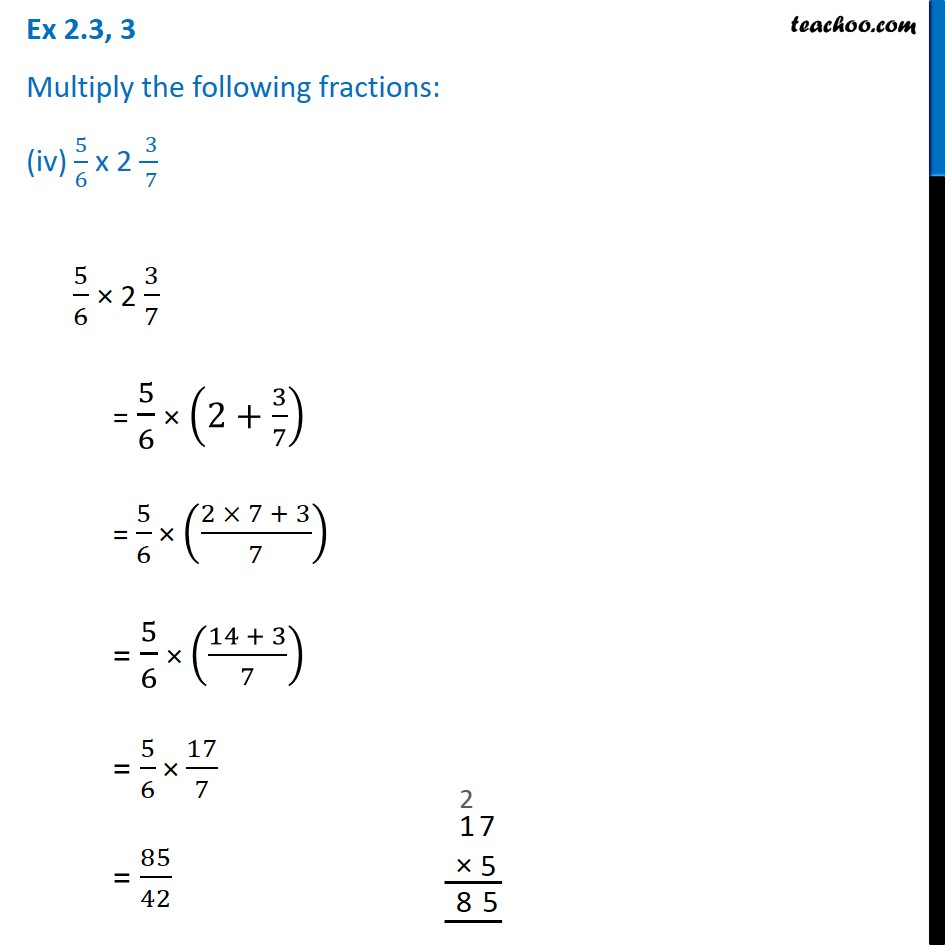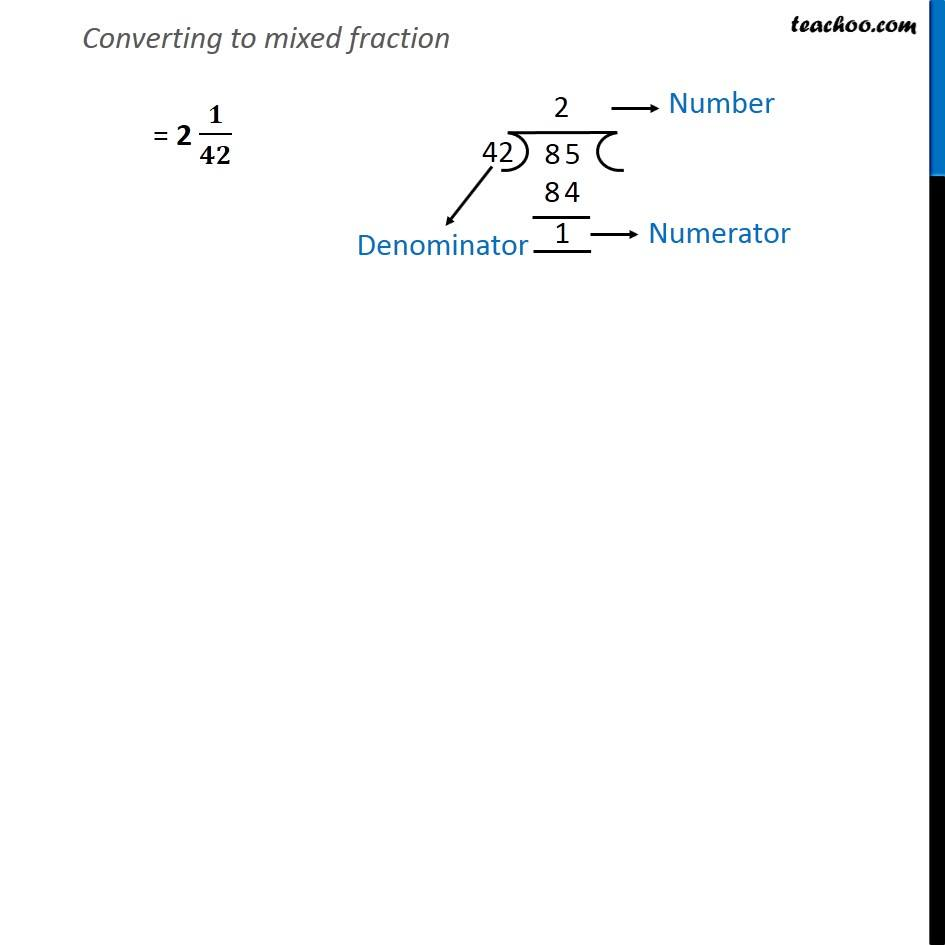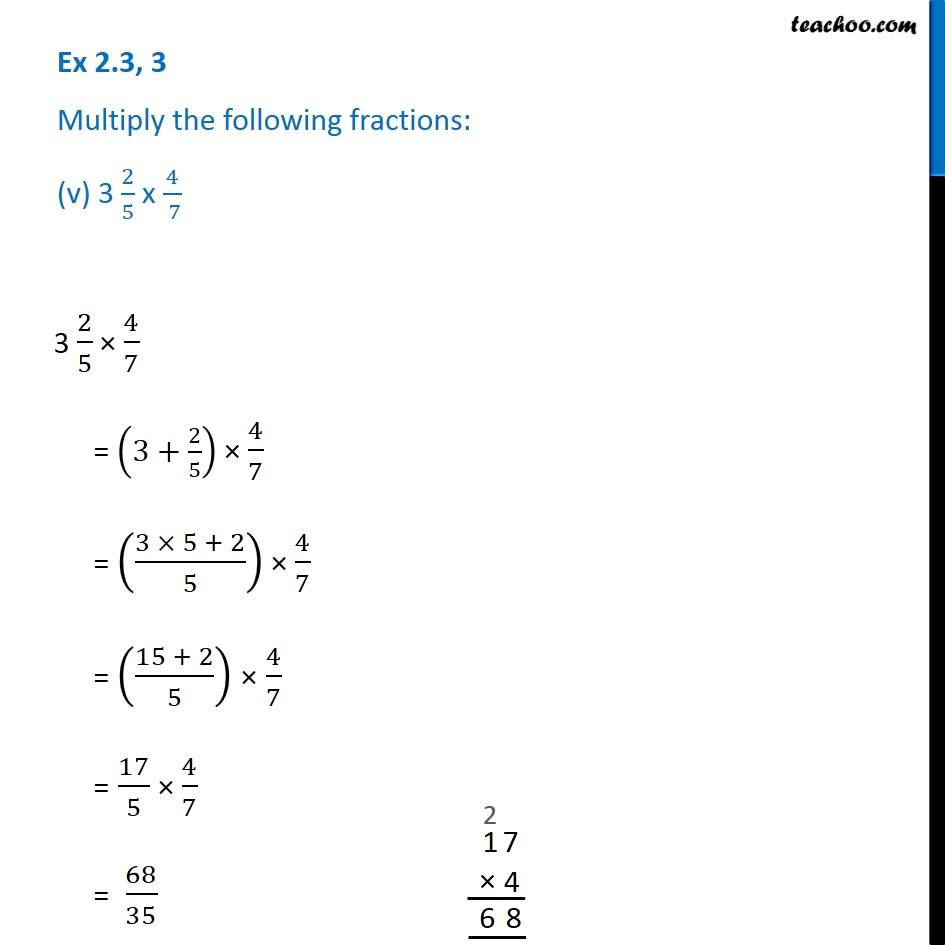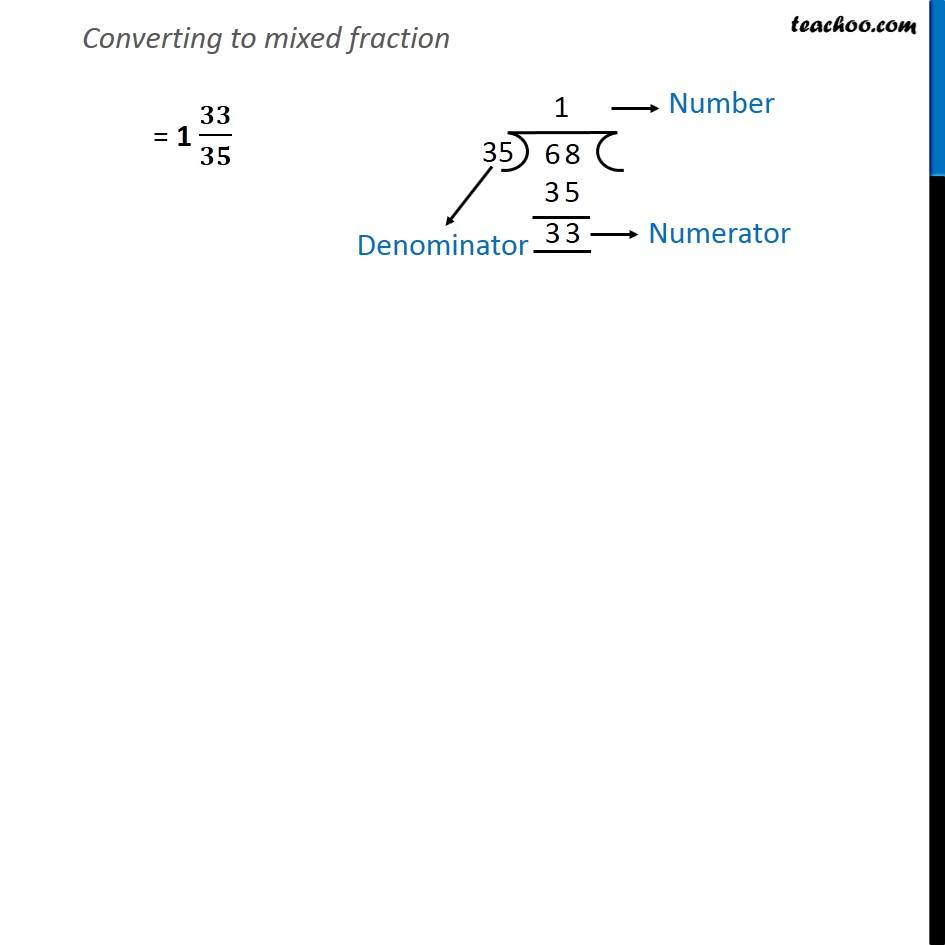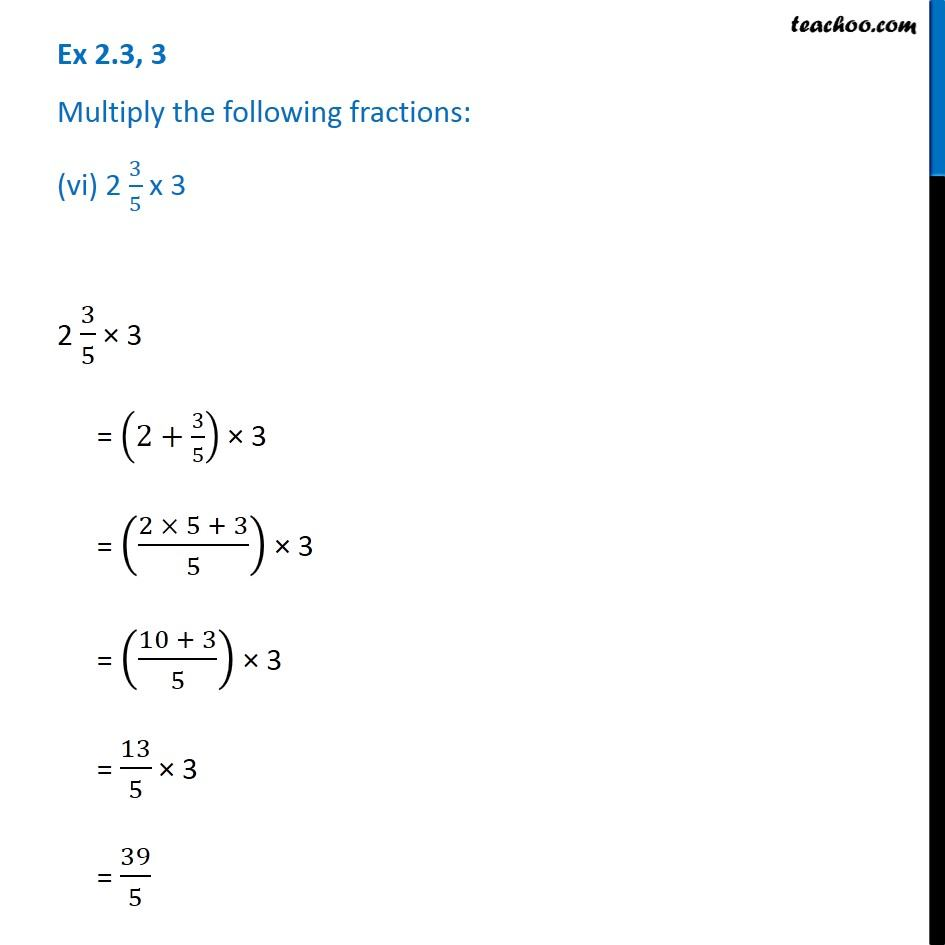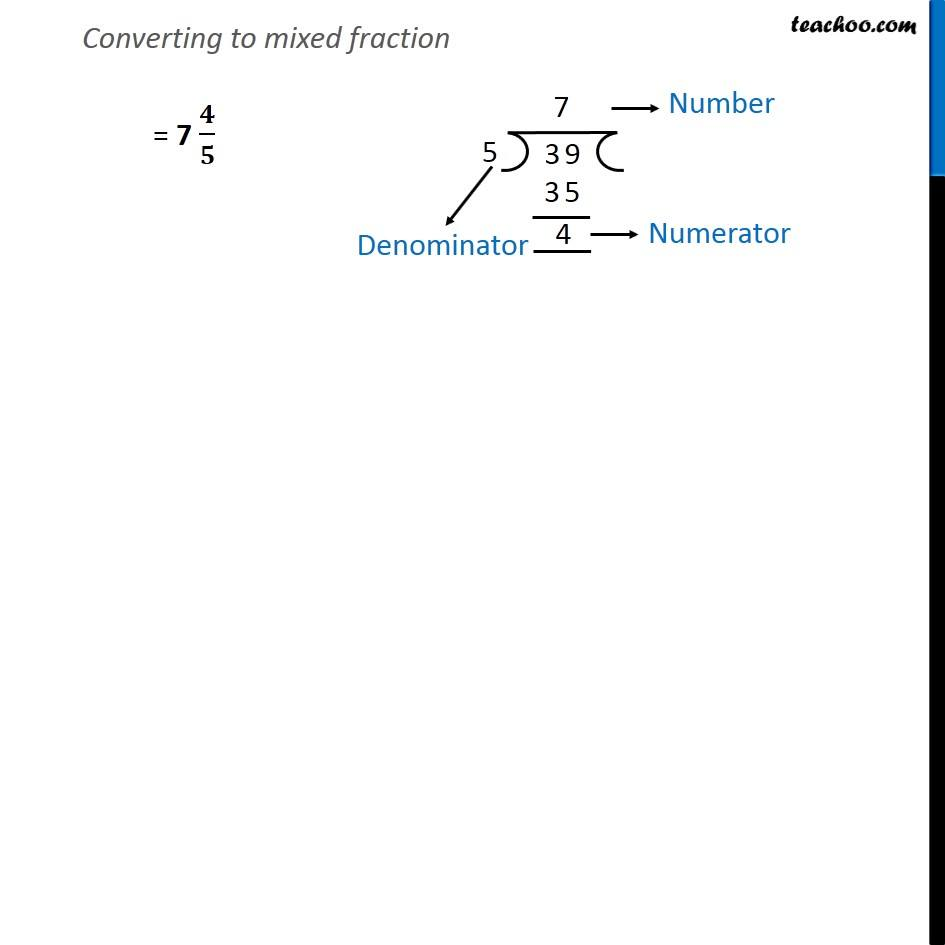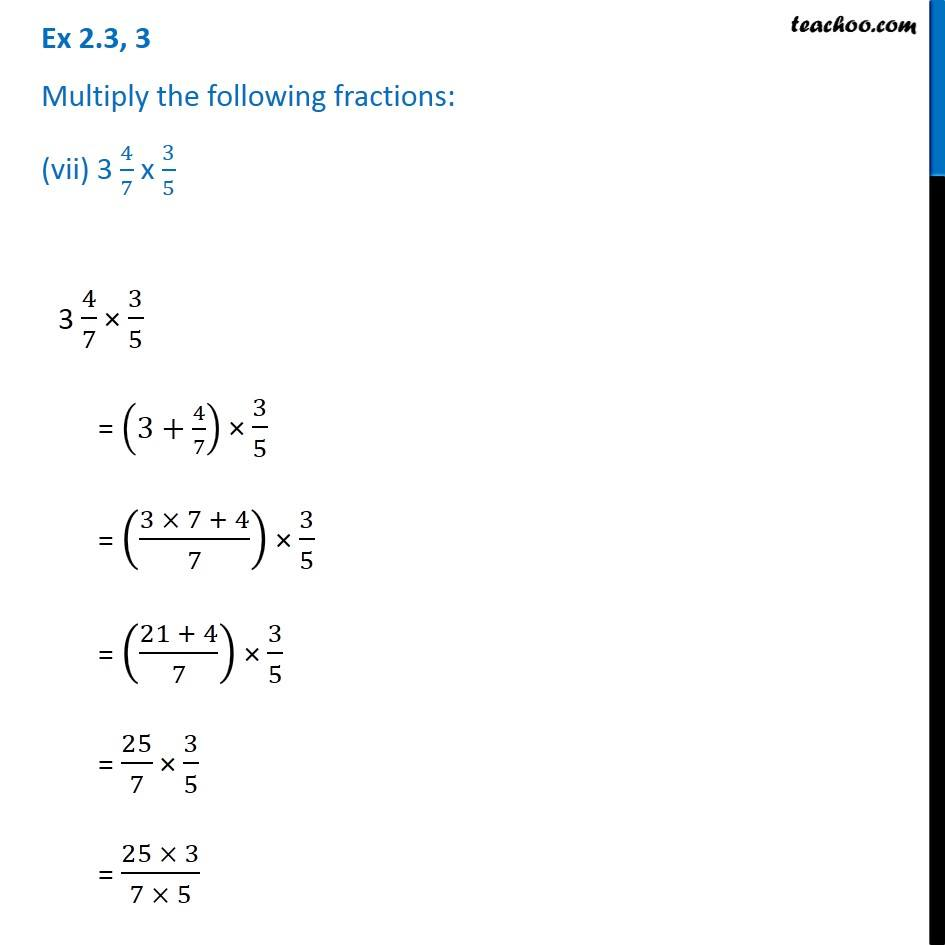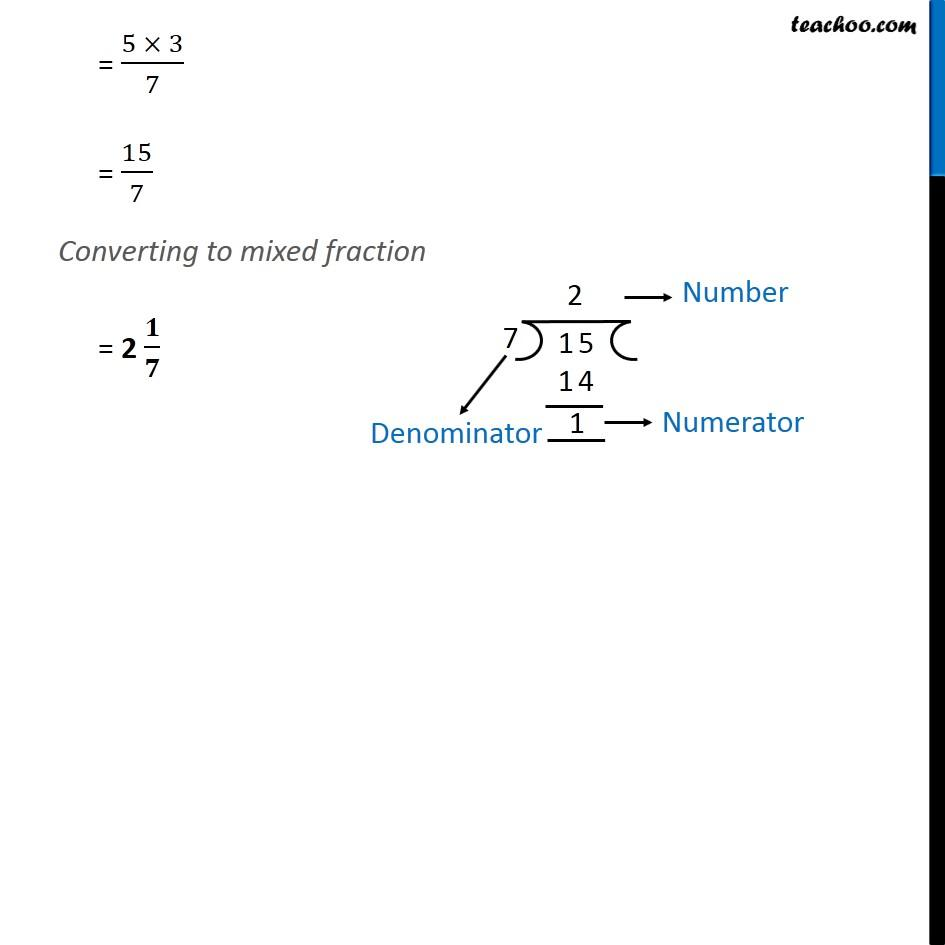Subscribe to our Youtube Channel - https://you.tube/teachoo

1. Chapter 2 Class 7 Fractions and Decimals
2. Concept wise
3. Multiplication of Fractions

Transcript

Ex 2.3, 3 Multiply the following fractions: (i) 2/5 x 5 1/4 2/5 × 5 1/4 = 2/5 × (5+1/4) = 2/5 × ((5 × 4 + 1 )/4) = 2/5 × ((20 + 1)/4) = 2/5 × 21/4 = (2 × 21)/(5 × 4) = 21/(5 × 2) = 21/10 Since Numerator > Denominator We will convert it into mixed fraction = 2 𝟏/𝟏𝟎 Ex 2.3, 3 Multiply the following fractions: (ii) 6 2/5 x 7/9 6 2/5 × 7/9 = (6+2/5) × 7/9 = ((6 × 5 + 2)/5) × 7/9 = ((30 + 2)/5) × 7/9 = 32/5 × 7/9 = (32 × 7)/(5 × 9) = 224/45 Since Numerator > Denominator We will convert it into mixed fraction = 4 𝟒𝟒/𝟒𝟓 Ex 2.3, 3 Multiply the following fractions: (iii) 3/2 x 5 1/3 3/2 × 5 1/3 = 3/2 × (5+1/3) = 3/2 × ((5 × 3 + 1)/3) = 3/2 × ((15 + 1)/3) = 3/2 × 16/3 = (3 × 16)/(2 × 3) = 16/2 = 8 Ex 2.3, 3 Multiply the following fractions: (iv) 5/6 x 2 ( 3)/( 7) 5/6 × 2 3/7 = 5/6 × (2+3/7) = 5/6 × ((2 × 7 + 3)/7) = 5/6 × ((14 + 3)/7) = 5/6 × 17/7 = 85/42 Converting to mixed fraction = 2 𝟏/𝟒𝟐 Ex 2.3, 3 Multiply the following fractions: (v) 3 2/5 x 4/( 7) 3 2/5 × 4/7 = (3+2/5) × 4/7 = ((3 × 5 + 2)/5) × 4/7 = ((15 + 2)/5) × 4/7 = 17/5 × 4/7 = 68/35 Converting to mixed fraction = 1 𝟑𝟑/𝟑𝟓 Ex 2.3, 3 Multiply the following fractions: (vi) 2 3/5 x 3 2 3/5 × 3 = (2+3/5) × 3 = ((2 × 5 + 3)/5) × 3 = ((10 + 3)/5) × 3 = 13/5 × 3 = 39/5 Converting to mixed fraction = 7 𝟒/𝟓 Ex 2.3, 3 Multiply the following fractions: (vii) 3 4/7 x 3/5 3 4/7 × 3/5 = (3+4/7) × 3/5 = ((3 × 7 + 4)/7) × 3/5 = ((21 + 4)/7) × 3/5 = 25/7 × 3/5 = (25 × 3)/(7 × 5) = (5 × 3)/7 = 15/7 Converting to mixed fraction = 2 𝟏/𝟕

Multiplication of Fractions